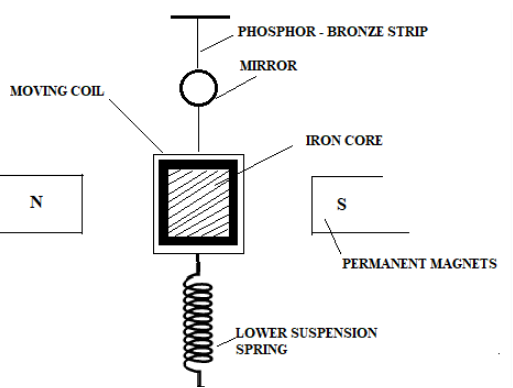# MCQ on Moving Coil Galvanometer

A moving coil galvanometer is an instrument which is used to measure electric currents. It is a sensitive electromagnetic device, which can measure low currents even of the order of a few microamperes.The moving coil galvanometer has a rectangular coil that has many turns and it is usually made of thinly insulated or fine copper wire that is wounded on a metallic frame. The coil is free to rotate about a fixed axis. A phosphor-bronze strip is connected to a movable torsion head. This is used to suspend the coil in a uniform magnetic field.

In order to improve the strength of the magnetic field and to make the field radial a soft iron core is placed inside the coil  The lower part of the coil is attached to a phosphor-bronze spring having a small number of turns. Binding screws are connected at the other end of the spring.

A steady angular deflection is produced by the spring to produce a counter torque which balances the magnetic torque. A plane mirror which is attached to the suspension wire, along with a lamp and scale arrangement is used to measure the deflection of the coil. Zero-point of the scale is at the centre.

The deflection or twist θ of the moving coil galvanometer is measured as the value indicated on a scale by a pointer which is connected to the suspension wire.

θ= ( nAB / k)I

Therefore, θ ∝ I

The quantity nAB / k is a constant for a given galvanometer.

Q1: The deflection θ is related to the electric current I in a galvanometer by the relation

1. I ∝ θ
2. I ∝ tan θ
3. I ∝ sin θ
4. I ∝ cos θ

Q2: A moving coil galvanometer carries a current I and the magnetic field B is radial. The coil has N number of turns and an effective area A. The torque acting on the coil of a moving coil galvanometer is given by

1. NA2B2I
2. NABI2
3. NABI
4. N2ABI

Q3: The sensitivity of a moving coil galvanometer can be increased by decreasing

1. The number of turns in the coil
2. The area of the coil
3. The magnetic field
4. The couple per unit twist of the suspension

Answer: (d) The couple per unit twist of the suspension

Q4: In ballistic galvanometer, the frame on which the coil is wound is non-metallic. It is

1. To avoid the production of induced e.m.f
2. To avoid the production of eddy currents
3. To increase the production of eddy currents
4. To increase the production of induced e.m.f

Answer: (b) To avoid the production of eddy currents

Q5: The reason the coil is bound over the metallic frame in moving coil galvanometer is

1. The metallic frame helps in oscillation
2. The metallic frame helps in making steady deflection without any oscillation
3. The metallic frame increases the magnetic field
4. None of the above

Answer: (b) The metallic frame helps in making steady deflection without any oscillation

Q6: The deflection in moving coil galvanometer is

1. Inversely proportional to the area of the coil
2. Directly proportional to the torsional constant
3. inversely proportional to the current flowing
4. Directly proportional to the number of turns of the coil

Answer: (d) Directly proportional to the number of turns of the coil

Q7: The pole pieces of the magnet used in a pivoted coil galvanometer are

1. Plane surfaces of a horse-shoe magnet
2. Cylindrical surfaces of a bar magnet
3. Plane surfaces of a bar magnet
4. Cylindrical surfaces of a horse-shoe magnet

Answer: (d) Cylindrical surfaces of a horse-shoe magnet

Q8: A current-carrying rectangular coil placed in a uniform magnetic field. In which orientation will the coil rotate?

1. In any orientation
2. The magnetic field is parallel to the plane of the coil
3. The magnetic field is at 450 with the plane of the coil
4. The magnetic field is perpendicular to the plane

Answer: (d) The magnetic field is perpendicular to the plane

Q9: What is the shape of a magnet in moving coil galvanometer to make the radial magnetic field?

1. Convex cylindrical magnet
2. Horse-shoe magnet
3. Concave cylindrical magnet
4. None